## 电荷重整化

### 真空极化与电荷重整化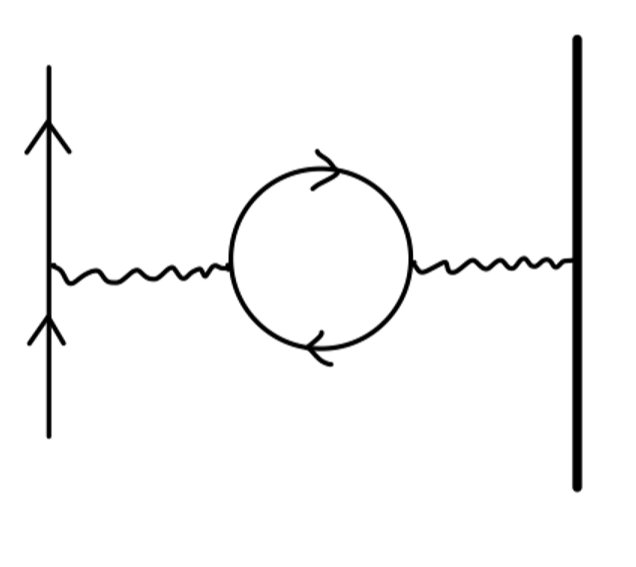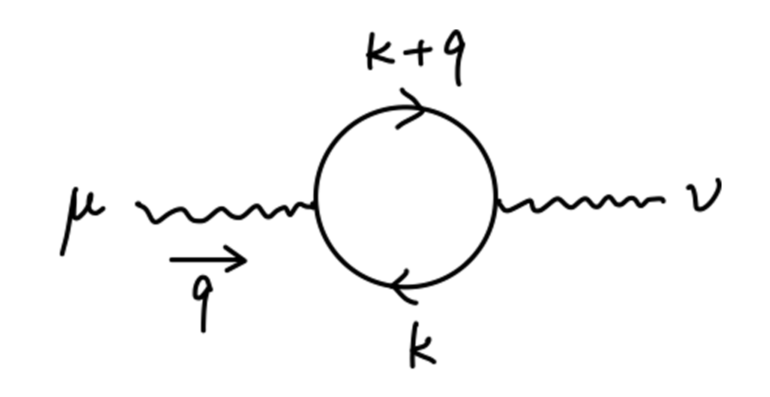$(-ie^2)(-1)\int\frac{d^4k}{(2\pi)^4}\mathrm{tr}[\gamma^{\mu}\frac{i}{\cancel{k}-m}\gamma^{\nu}\frac{i}{\cancel{k} + \cancel{q}-m}] \equiv i\Pi_2^{\mu\nu}(q)\tag{1}$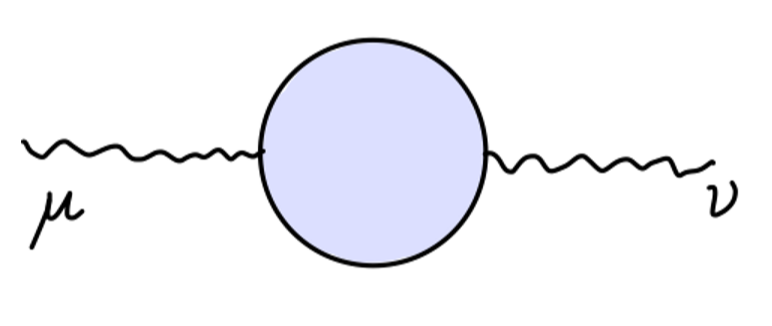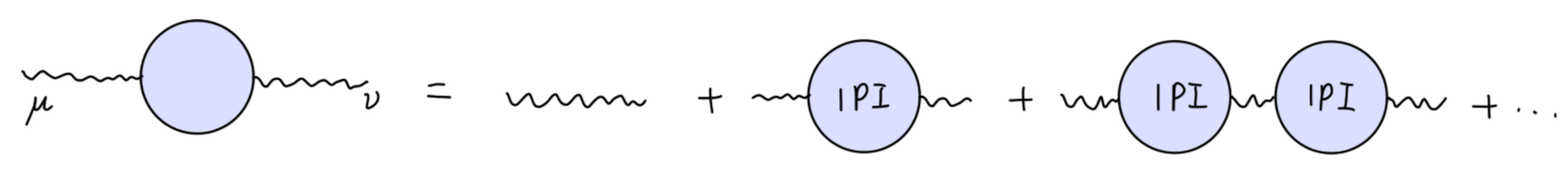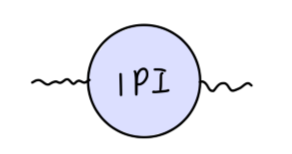$i\Pi^{\mu\nu}(q)$

$(1)$ 式中，我们之所以将 $\mathcal{O}(\alpha)$ 阶真空极化图的贡献称之为 $\Pi_2$，是因为它包含了 $e^2$，是一个对真空极化的关于 $e$ 的二阶贡献。

$q_{\mu}\Pi^{\mu\nu} = q_{\nu}\Pi^{\mu\nu} = 0$

$\Pi^{\mu\nu}(q) = (q^2g^{\mu\nu} - q^{\mu}q^{\nu})\Pi(q^2)\tag{2}$

\begin{aligned} &\frac{-ig_{\mu\nu}}{q^2} + \frac{-ig_{\mu\rho}}{q^2}[i(q^2g^{\rho\sigma} - q^{\rho}q^{\sigma})\Pi(q^2)]\frac{-ig_{\sigma\nu}}{q^2} + \cdots\\ \end{aligned}\tag{3}

$\Delta_{\nu}^{\rho} \equiv \delta_{\nu}^{\rho} - q^{\rho}q_{\nu}/q^2$。注意到：

\begin{aligned} \Delta_{\sigma}^{\rho}\Delta_{\nu}^{\sigma} &= (\delta_{\sigma}^{\rho} - q^{\rho}q_{\sigma}/q^2)(\delta_{\nu}^{\sigma} - q^{\sigma}q_{\nu}/q^2)\\ &= \delta^{\rho}_{\nu} - 2q^{\rho}q_{\nu}/q^2 + q^{\rho}q_{\nu}q^{\sigma}q_{\sigma}/q^4\\ &= \delta^{\rho}_{\nu} - q^{\rho}q_{\nu}/q^2 = \Delta^{\rho}_{\nu}\\ \end{aligned}\tag{4}

\begin{aligned} &\frac{-ig_{\mu\nu}}{q^2} + \frac{-ig_{\mu\rho}}{q^2}\Delta^{\rho}_{\nu}\Pi(q^2) + \frac{-ig_{\mu\rho}}{q^2}\Delta^{\rho}_{\sigma}\Delta^{\sigma}_{\nu}\Pi^2(q^2) + \cdots\\ =& \frac{-ig_{\mu\nu}}{q^2} + \frac{-ig_{\mu\rho}}{q^2}\Delta^{\rho}_{\nu}\Pi(q^2) + \frac{-ig_{\mu\rho}}{q^2}\Delta^{\rho}_{\nu}\Pi^2(q^2) + \cdots\\ =& \frac{-ig_{\mu\nu}}{q^2} + \frac{-ig_{\mu\rho}}{q^2}\Delta^{\rho}_{\nu}(\Pi(q^2)+\Pi^2(q^2)+\cdots)\\ =& \frac{-ig_{\mu\nu}}{q^2} + \frac{-ig_{\mu\rho}}{q^2}(\delta_{\nu}^{\rho} - \frac{q^{\rho}q_{\nu}}{q^2})\frac{\Pi(q^2)}{1-\Pi(q^2)}\\ =& \frac{-i}{q^2(1-\Pi(q^2))}(g_{\mu\nu}-\frac{q_{\mu}q_{\nu}}{q^2}) + \frac{-i}{q^2}(\frac{q_{\mu}q_{\nu}}{q^2}) \end{aligned}\tag{5}

$\frac{-ig_{\mu\nu}}{q^2(1-\Pi(q^2))}\tag{6}$

$\frac{1}{1-\Pi(0)} \equiv Z_3\tag{7}$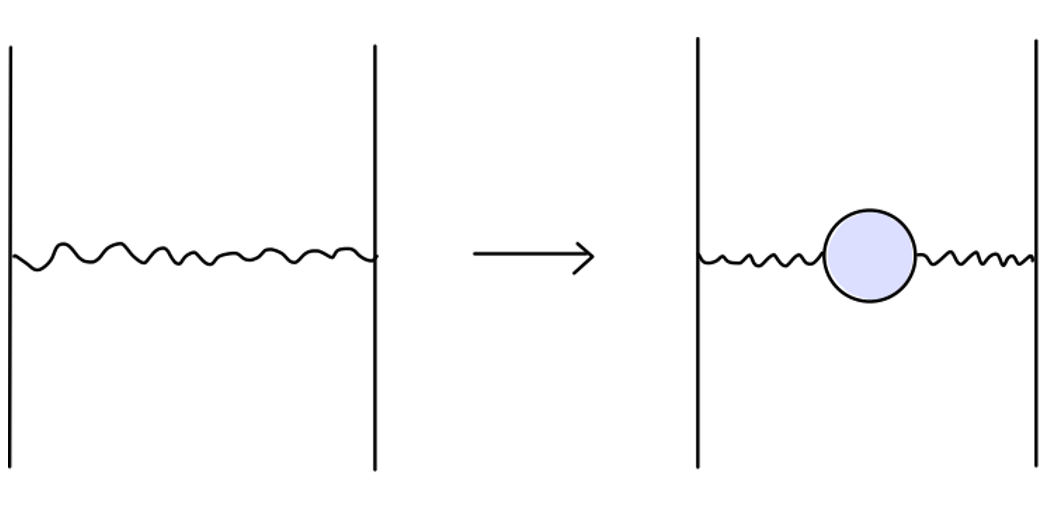$\cdots \frac{e^2g_{\mu\nu}}{q^2}\cdots \rightarrow \cdots \frac{Z_3 e^2g_{\mu\nu}}{q^2}\cdots$

$e \rightarrow \sqrt{Z_3}e$

$(physical\ charge) = e = \sqrt{Z_3}e_0 = \sqrt{Z_3}\cdot(bare\ charge)$

$\Pi(q^2) \approx \Pi_2(q^2)$

\begin{aligned} \frac{-ig_{\mu\nu}}{q^2}(\frac{e_0^2}{1-\Pi(q^2)}) &=\frac{-ig_{\mu\nu}}{q^2}(\frac{e_0^2}{1-\Pi(0)})(\frac{1-\Pi(0)}{1-\Pi(q^2)})\\ &\underset{\mathcal{O}(\alpha)}{=}\frac{-ig_{\mu\nu}}{q^2}(\frac{e^2}{1-[\Pi(q^2)-\Pi(0)]})\\ \end{aligned}

$\alpha_0 \rightarrow \alpha_{eff}(q^2) = \frac{e_0^2/4\pi}{1-\Pi(q^2)} \underset{\mathcal{O}(\alpha)}{=} \frac{\alpha}{1-[\Pi_2(q^2)-\Pi_2(0)]}$

### $\Pi_2$ 的计算

\begin{aligned} i\Pi_2^{\mu\nu}(q) &= -(-ie)^2\int \frac{d^4k}{(2\pi)^4}\mathrm{tr}[\gamma^{\mu}\frac{i(\cancel{k}+m)}{k^2-m^2}\gamma^{\nu}\frac{i(\cancel{k}+\cancel{q}+m)}{(k+q)^2-m^2}]\\ &= -e^2 \int \frac{d^4k}{(2\pi)^4}\frac{\mathrm{tr}[\gamma^{\mu}(\cancel{k}+m)\gamma^{\nu}(\cancel{k}+\cancel{q}+m)]}{(k^2-m^2)((k+q)^2-m^2)}\\ &= -4e^2\int \frac{d^4k}{(2\pi)^4}\frac{k^{\mu}(k+q)^{\nu}+k^{\nu}(k+q)^{\mu}-g^{\mu\nu}(k\cdot(k+q)-m^2)}{(k^2-m^2)((k+q)^2-m^2)} \end{aligned}

\begin{aligned} \frac{1}{(k^2-m^2)((k+q)^2-m^2)} &= \int_{0}^{1}dx \frac{1}{(k^2+2xk\cdot q + xq^2 -m^2)^2}\\ &\underset{l = k + xq}{=} \int_{0}^{1}dx \frac{1}{l^2 + x(1-x)q^2-m^2} \end{aligned}

\begin{aligned} &(l-xq)^{\mu}(l+(1-x)q)^{\nu} + (l-xq)^{\nu}(l+(1-x)q)^{\mu} - g^{\mu\nu}((l-xq)\cdot(l+(1-x)q)-m^2)\\ =& 2l^{\mu}l^{\nu} -g^{\mu\nu}l^2 - 2x(1-x)q^{\mu}q^{\nu} + g^{\mu\nu}(m^2 + x(1-x)q^2) + (terms\ linear\ in\ l) \end{aligned}

\begin{aligned} i\Pi_2^{\mu\nu}(q) &= -4ie^2\int_{0}^{1}dx\int \frac{d^4l_E}{(2\pi)^4} \frac{-2l_E^{\mu}l_E^{\nu} +g^{\mu\nu}l_E^2 - 2x(1-x)q^{\mu}q^{\nu} + g^{\mu\nu}(m^2 + x(1-x)q^2)}{(l_E^2 + \Delta)^2}\\ &= -4ie^2\int_{0}^{1}dx\int \frac{d^4l_E}{(2\pi)^4} \frac{-\frac{1}{2}g^{\mu\nu}l_E^2 +g^{\mu\nu}l_E^2 - 2x(1-x)q^{\mu}q^{\nu} + g^{\mu\nu}(m^2 + x(1-x)q^2)}{(l_E^2 + \Delta)^2}\\ \end{aligned}

$\Delta = m^2 - x(1-x)q^2$

$i\Pi^{\mu\nu}_2(q) \propto e^2\Lambda^2g^{\mu\nu}$

#### 维度正规化

$\int \frac{d^dl_E}{(2\pi)^d}\frac{1}{(l_E^2+\Delta)^2} = \int \frac{d\Omega_d}{(2\pi)^d}\cdot \int_{0}^{\infty}dl_E \frac{l_E^{d-1}}{(l_E^2+\Delta)^2}\tag{8}$

\begin{aligned} (\sqrt{\pi})^d &= (\int dx e^{-x^2})^d\\ &= \int d^dx \exp(-\sum_{i=1}^d x_i^2)\\ &= \int d\Omega_d \int_{0}^{\infty}dx x^{d-1}e^{-x^2}\\ &= (\int d\Omega_d)\cdot \frac{1}{2}\int_{0}^{\infty}dx^2 (x^2)^{\frac{d}{2}-1}e^{-(x^2)}\\ &= (\int d\Omega_d) \cdot \frac{1}{2}\Gamma(\frac{d}{2}) \end{aligned}

$\int d\Omega_d = \frac{2\pi^{d/2}}{\Gamma(d/2)}\tag{9}$

\begin{aligned} \int_{0}^{\infty}dl \frac{l^{d-1}}{(l^2+\Delta)^2} &= \frac{1}{2}\int_{0}^{\infty}d(l^2)\frac{(l^2)^{\frac{d}{2}-1}}{(l^2+\Delta)^2}\\ &= \frac{1}{2} (\frac{1}{\Delta})^{2-\frac{d}{2}}\int_{0}^{1}dx x^{1-\frac{d}{2}}(1-x)^{\frac{d}{2}-1}\\ &= \frac{1}{2} (\frac{1}{\Delta})^{2-\frac{d}{2}} \frac{\Gamma(\frac{d}{2})\Gamma(2-\frac{d}{2})}{\Gamma(2)}\\ \end{aligned}\tag{10}

$\int_{0}^{1}x^{\alpha-1}(1-x)^{\beta-1} = B(\alpha,\beta) = \frac{\Gamma(\alpha)\Gamma(\beta)}{\Gamma(\alpha+\beta)}$

\begin{aligned} \int \frac{d^dl_E}{(2\pi)^d}\frac{1}{(l_E^2+\Delta)^2} &=\frac{1}{(2\pi)^d} \frac{2\pi^{d/2}}{\Gamma(d/2)}\cdot \frac{1}{2} (\frac{1}{\Delta})^{2-\frac{d}{2}} \frac{\Gamma(\frac{d}{2})\Gamma(2-\frac{d}{2})}{\Gamma(2)}\\ &= \frac{1}{(4\pi)^{d/2}}\frac{\Gamma(2-\frac{d}{2})}{\Gamma(2)}(\frac{1}{\Delta})^{2-\frac{d}{2}} \end{aligned}\tag{11}

$\Gamma(2-\frac{d}{2}) = \Gamma(\frac{\epsilon}{2}) = \frac{2}{\epsilon} - \gamma + \mathcal{O}(\epsilon)$

\begin{aligned} \int \frac{d^dl_E}{(2\pi)^d}\frac{1}{(l_E^2+\Delta)^2} &= \frac{1}{(4\pi)^2}\Gamma(\frac{\epsilon}{2})(\frac{4\pi}{\Delta})^{\epsilon/2}\\ &\underset{\epsilon\rightarrow 0}{\longrightarrow}\frac{1}{(4\pi)^2}(\frac{2}{\epsilon} - \gamma + \mathcal{O}(\epsilon))(1 + \frac{\epsilon}{2} \log \frac{4\pi}{\Delta} + \mathcal{O}(\epsilon))\\ &= \frac{1}{(4\pi)^2}(\frac{2}{\epsilon} -\log\Delta - \gamma + \log (4\pi) + \mathcal{O}(\epsilon)) \end{aligned}

$\int \frac{d^4 l_E}{(2\pi)^4}\frac{1}{(l_E^2+\Delta)^2} \underset{\Lambda \rightarrow \infty}{\longrightarrow} \frac{1}{(4\pi)^2}(\log \frac{x\Lambda^2}{\Delta} + \mathcal{O}(\Lambda^{-1}))$

\begin{aligned} \int \frac{d^dl_E}{(2\pi)^d}\frac{1}{(l_E^2+\Delta)^n} &= \frac{1}{(4\pi)^{d/2}}\frac{\Gamma(n-\frac{d}{2})}{\Gamma(n)}(\frac{1}{\Delta})^{n-\frac{d}{2}}\\ \int \frac{d^dl_E}{(2\pi)^d}\frac{l_E^2}{(l_E^2+\Delta)^n} &= \frac{1}{(4\pi)^{d/2}}\frac{d}{2}\frac{\Gamma(n-\frac{d}{2}-1)}{\Gamma(n)}(\frac{1}{\Delta})^{n-\frac{d}{2}-1}\\ \end{aligned}

$d$ 维时空中有：

$g^{\mu\nu}g_{\mu\nu} = d$

$l^{\mu}l^{\nu} \rightarrow \frac{1}{d}l^2g^{\mu\nu}$

$\{\gamma^{\mu},\gamma^{\nu}\},\quad \mathrm{tr} = 4$

\begin{aligned} \gamma^{\mu}\gamma^{\nu}\gamma_{\mu} &= -(2-\epsilon)\gamma^{\nu}\\ \gamma^{\mu}\gamma^{\nu}\gamma^{\rho}\gamma_{\mu} &= 4g^{\nu\rho} - \epsilon\gamma^{\nu}\gamma^{\rho}\\ \gamma^{\mu}\gamma^{\nu}\gamma^{\rho}\gamma^{\sigma}\gamma_{\mu} &= -2\gamma^{\sigma}\gamma^{\rho}\gamma^{\nu} + \epsilon\gamma^{\nu}\gamma^{\rho}\gamma^{\sigma}\\ \end{aligned}

#### 使用维度正规化计算 $\Pi_2$

\begin{aligned} \int \frac{d^dl_E}{(2\pi)^d}\frac{(-\frac{2}{d}+1)g^{\mu\nu}l_E^2}{(l_E^2+\Delta)^2} &= -\frac{1}{(4\pi)^{d/2}}(1-\frac{d}{2})\Gamma(1-\frac{d}{2})(\frac{1}{\Delta})^{1-\frac{d}{2}}g^{\mu\nu}\\ &= \frac{1}{(4\pi)^{d/2}}\Gamma(2-\frac{d}{2})(\frac{1}{\Delta})^{2-\frac{d}{2}}(-\Delta g^{\mu\nu})\\ \end{aligned}

\begin{aligned} i\Pi_2^{\mu\nu}(q) &= -4ie^2\int_{0}^{1}dx \frac{1}{(4\pi)^{d/2}} \frac{\Gamma(2-\frac{d}{2})}{\Delta^{2-d/2}}\\ &\quad\cdot (-\Delta g^{\mu\nu} - 2x(1-x)q^{\mu}q^{\nu} + g^{\mu\nu}(m^2+x(1-x)q^2))\\ &= -4ie^2\int_{0}^{1}dx \frac{1}{(4\pi)^{d/2}} \frac{\Gamma(2-\frac{d}{2})}{\Delta^{2-d/2}}\cdot 2x(1-x)(q^2g^{\mu\nu}-q^{\mu}q^{\nu})\\ &= (q^2g^{\mu\nu}-q^{\mu}q^{\nu})\cdot i\Pi_2(q^2)\\ \end{aligned}

\begin{aligned} \Pi_2(q^2) &= \frac{-8e^2}{(4\pi)^{d/2}}\int_{0}^{1}dx x(1-x) \frac{\Gamma(2-\frac{d}{2})}{\Delta^{2-d/2}}\\ &\underset{d\rightarrow 4}{\longrightarrow} \frac{-8e^2}{(4\pi)^2} \int_{0}^{1}dxx(1-x)(\frac{2}{\epsilon} -\log\Delta - \gamma + \log (4\pi))\\ &= -\frac{2\alpha}{\pi} \int_{0}^{1}dx x(1-x)(\frac{2}{\epsilon} -\log\Delta - \gamma + \log (4\pi))\\ \end{aligned}

$\frac{e^2-e_0^2}{e^2_0} = \delta Z_3 \underset{\mathcal{O}(\alpha)}{=}\Pi_2(0) \approx -\frac{2\alpha}{3\pi\epsilon}$

\begin{aligned} \hat{\Pi}_2(q^2) &= \Pi_2(q^2) - \Pi_2(0)\\ &= -\frac{2\alpha}{\pi} \int_{0}^{1}dx x(1-x)\log(\frac{m^2}{m^2-x(1-x)q^2}) \end{aligned}\tag{12}

#### $\Pi_2$ 的分析

$m^2 -\frac{1}{4}q^2$

$x\in(\frac{1}{2}-\frac{1}{2}\beta,\frac{1}{2}+\frac{1}{2}\beta),\quad \beta = \sqrt{1-\frac{4m^2}{q^2}}$

\begin{aligned} \mathrm{Im}[\hat{\Pi}_2(q^2\pm i\epsilon)] &= -\frac{2\alpha}{\pi}\int_{\frac{1}{2}-\frac{1}{2}\beta}^{\frac{1}{2}+\frac{1}{2}\beta} dx x(1-x)\mathrm{Im}(-\log(m^2-x(1-x)(q^2\pm i\epsilon)))\\ &= -\frac{2\alpha}{\pi}(\pm \pi)\int_{\frac{1}{2}-\frac{1}{2}\beta}^{\frac{1}{2}+\frac{1}{2}\beta} dx x(1-x)\\ &= \mp \frac{\alpha}{3}\sqrt{1-\frac{4m^2}{q^2}}(1+\frac{2m^2}{q^2})\\ \end{aligned}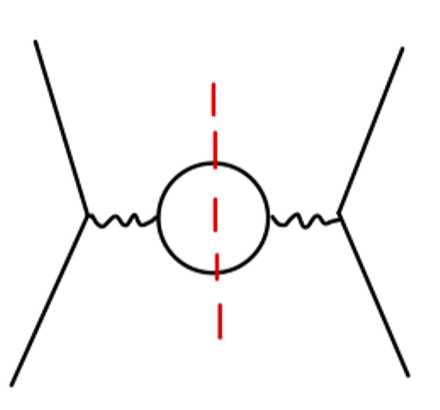$V(\bm{x}) = \frac{ie^2}{(2\pi)^2r}\int_{-\infty}^{\infty}dQ \frac{Qe^{iQr}}{Q^2+\mu^2}[1+\hat{\Pi}_2(-Q^2)]\tag{13}$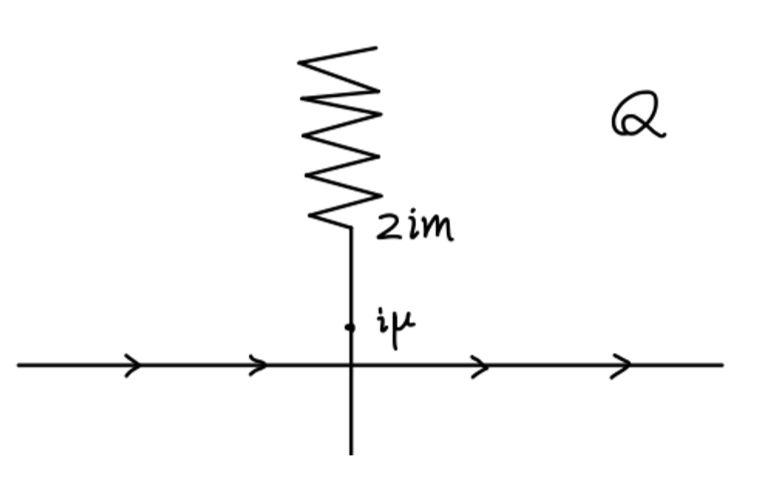$V = \frac{\alpha}{r}$

\begin{aligned} \delta V(r) &= \frac{-e^2}{(2\pi)^2r}\cdot 2\int_{2m}^{\infty}dq\frac{e^{-qr}}{q}\mathrm{Im}[\hat{\Pi}_2(q^2-i\epsilon)]\\ &= -\frac{\alpha}{r}\frac{2}{\pi}\cdot\int_{2m}^{\infty}dq\frac{e^{-qr}}{q}\frac{\alpha}{3}\sqrt{1-\frac{4m^2}{q^2}}(1+\frac{2m^2}{q^2}) \end{aligned}\tag{14}

$r \gg 1/m$ 时，该积分主要由 $q\approx 2m$ 的区域贡献。做代换 $t = q-2m$，可以近似得到：

\begin{aligned} \delta V(r) &\approx -\frac{\alpha}{r}\frac{2}{\pi}\cdot\int_{0}^{\infty}dt \frac{e^{-(t+2m)r}}{2m}\frac{\alpha}{3}\sqrt{\frac{t}{m}}(\frac{3}{2})\\ &=-\frac{\alpha}{r}\cdot\frac{\alpha}{4\sqrt{\pi}}\frac{e^{-2mr}}{(mr)^{3/2}} \tag{15} \end{aligned}

$V(r) = \frac{\alpha}{r}(1 + \frac{\alpha}{4\sqrt{\pi}}\frac{e^{-2mr}}{(mr)^{3/2}} + \cdots) \tag{16}$

\begin{aligned} \hat{\Pi}_2(q^2) &\approx \frac{2\alpha}{\pi}\int_{0}^{1}dxx(1-x)(\log(-\frac{q^2}{m^2}) + \log(x(1-x)) + \mathcal{O}(\frac{m^2}{q^2}))\\ &= \frac{\alpha}{3\pi}[\log(-\frac{q^2}{m^2}) -\frac{5}{3} + \mathcal{O}(\frac{m^2}{q^2})] \end{aligned}

$\alpha_{eff}(q^2) = \frac{\alpha}{1-\frac{\alpha}{3\pi}\log(-\frac{q^2}{Am^2})}$